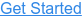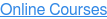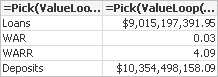Where do you want to go?# Synthetic Dimensions with Better Performance than Valuelists

by Speros Kokenes, on Jul 8, 2013 9:53:29 AM

The QlikView Design Blog recently posted about using valuelists and valueloops as calculated dimensions to create synthetic dimensions. As they demonstrate, this can be used to create tables of KPIs, among other charts.

I have found this approach to be extremely useful in the past and frequently employed it. However, I was recently at a client with a large data set and was doing some performance tuning when I found an approach to synthetic dimension creation that I found to improve performance drastically over the use of valuelists and valueloops.

Instead of using a valuelist or valueloop with a calculated dimension, you can create a field in your data model with dual values that correspond with the label and row number of the field value. This can be placed in an island table that does not connect to your data model. Then this can be used instead of the calculated dimension in your charts.

I created an example using a set of demo data I have for the banking industry. My goal was to create a KPI table with 4 metrics: Loans, Weighted Average Rate, Weighted Average Risk Rating, and Deposits. I created separate documents where I created the chart twice: once via valueloop, and once via dual dimension.

Test Case 1: ValueLoopThe table above contains the following attributes:

Dimension: =valueloop(1,4)

Expression1: =Pick(ValueLoop(1,4),'Loans','WAR','WARR','Deposits')

Expression2: =Pick(ValueLoop(1,4),money(sum([Loan Principal Balance])),num(sum([Loan Current Interest Rate]*[Loan Principal Balance])/sum([Loan Principal Balance]),'#.##'),num(sum([Loan Risk Rating]*[Loan Principal Balance])/sum([Loan Principal Balance]),'#.##'),money(sum([Deposit Balance])))

The dimension is hidden in this example. I prefer this approach typically because it allows for line items to be created with the same labels. This is useful when trying to create a list of KPI's with submetrics, like:

 Loans X,XXX Weighted X.XX Deposits X,XXX Weighted X.XX

Test Case 2: Dual DimensionThis table contains the following attributes:

Dimension: %DualTest

Expression: =Pick(%DualTest,money(sum([Loan Principal Balance])),num(sum([Loan Current Interest Rate]*[Loan Principal Balance])/sum([Loan Principal Balance]),'#.##'),num(sum([Loan Risk Rating]*[Loan Principal Balance])/sum([Loan Principal Balance]),'#.##'),money(sum([Deposit Balance])))

The %DualTest field was created with the following script:

[%DualDimTest]:

AutoGenerate(1);

AutoGenerate(1);

AutoGenerate(1);

AutoGenerate(1);

In these load statements, we use the dual function to simultaneously assign a text value and a numeric value to the field %DualTest. This allows us to display it as text in the dimension of the chart while referencing its numeric value in the expression of the chart.

Test Results

To test each chart, I saved each document on a blank sheet. Then, I closed QlikView completely and re-opened the document. From there, I navigated to the sheet containing the chart and checked the Calc Time in Sheet Properties > Objects. I repeated these steps 5 times for each chart. The results, in milliseconds:

 ValueList Dual Dim Trial 1 203 78 Trial 2 234 47 Trial 3 219 63 Trial 4 249 63 Trial 5 234 63 Avg 227.8 62.8

The valueloop chart performed almost 4x worse than the chart using the dual dimension. The 1st test case does have an extra expression, so I also tried the chart without the extra expression to add the labels. This decreased the average down to ~200 ms, which is still much worse than the dual dimension approach.

This test indicates to me that when possible, an isolated dimension should be used instead of valuelists to create synthetic dimensions.

-Speros